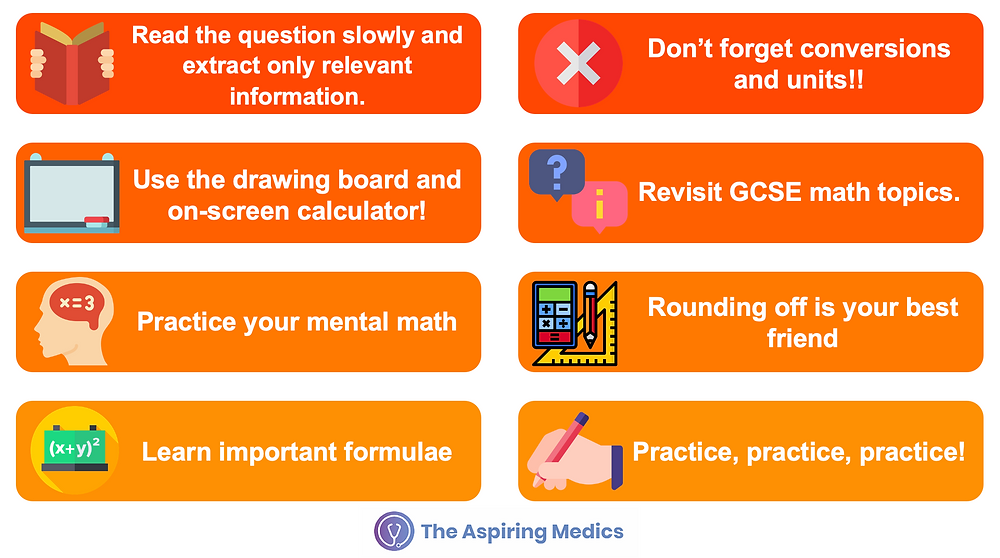Search
•The Aspiring Medics

# 8 Top Tips for Quantitative Reasoning

In this series of articles, we take you through the medicine application process for UK universities. Through this series of comprehensive blogs, you will know absolutely everything you need to stand out from the crowd during the application process.

The Quantitative Reasoning section is the third section of the UCAT exam. This section focuses on testing your numerical problem-solving abilities and thus involves evaluating numerical data sets by answering multiple-choice questions. Questions include the use of graphs, charts, shapes, and tables with data. They may also include percentages, fractions, decimals and ratios.

The most important thing to keep in mind about this section is that its focus is problem-solving not testing your mathematical abilities (this has directly been mentioned on the UCAT website). Often, students are afraid of this section because they don't do A-Level Maths and have forgotten mathematical concepts studied at GCSE. This section, however, is focused on figuring out how to solve a question - what formulae to use, what values to use and so on. Once you figure out the method, the actual calculations aren't that difficult - and you even have a calculator to help you.

This article is written by one of our top tutors, Arisma, who scores 3080 in the 2019 UCAT, putting her in the top 1% of the world. She scored 800 in the Verbal Reasoning section.

In this article, we take you through our Top 8 Tips to score high in the Quantitative Reasoning section.### 📖 Read the Questions Carefully

Quantitative Reasoning questions often include irrelevant mathematical details. Make sure to take time out, in the beginning, to extract relevant information to avoid mistakes.

### ❌ Don't Forget Units and Conversions

Make sure the unit in your answer matches the unit in the question.### 🖋 Use the Drawing Board and Calculator

The online calculator provided by the UCAT exam is very different to what you will be used to. Although mental maths should get you through much of the exam, there will be a few times where the calculator may be necessary, and being confident in using the calculator quickly will save precious seconds. We recommend practising using the calculator for more complicated calculations as part of your QR training, so it accurately simulates the exam conditions. We would also recommend familiarizing yourself with the memory function and other shortcuts (such as not typing the 0 before a decimal point) which may make you as fast as possible.

### 🧮 Revisit GCSE Math Topics

• Times tables up to 12 (and multiplying larger numbers by single-digit numbers)

• Adding and subtracting up to 4-digit numbers

• Unit conversions (milli to micro to nano, kilo to mega to giga etc.)

• Convert Fractions, decimals and percentages, and calculations involving these. (Top Tip: these calculations can be done either way round, e.g. 40% of 25 is the same as 25% of 40, and the latter is much easier to deal with mentally)

• Working with different types of average (mean, median, mode and ranges)

• Speed = Distance/Time calculations

• Rearrangement of formulas and equations, and basic algebra

• Areas and perimeter of triangles, rectangles, circles and parallelograms

• Volume and surface area of spheres, cubes/cuboids and cylinder

• Interpreting information from complex graphs and tables

• Rounding where applicable (detail further down)

• Practising these so that you are able to do simple calculations involving them quickly and without the need for writing or using a calculator will help you keep to time and allow you to score higher.

### 🧠 Practice your Mental Math

The QR section relies very heavily on mental arithmetic, as you are forbidden to bring a handheld calculator into the exam, and the on-screen calculator is very slow and time-consuming, not to mention difficult to use.

After at least 1 year of A-Levels, you will be very reliant on your calculator for any maths work you're doing, meaning your mental maths will now be surprisingly bad, especially if you haven’t been taking a maths A-Level. Relying on the UCAT Calculator for anything that isn’t absolutely necessary, will drain your precious time. The answer to this, sadly, is spending hours and hours speeding yourself up. Spend as much time as possible practising maths without a calculator, because the more you do, the quicker and easier it will become.

### 🔵 Rounding Off is your Best Friend

Sometimes a question will have numbers that are difficult to work with and require frustrating use of the calculator.

In these questions, it may be useful to take a glance at the answer options, and if they are far apart, doing a calculation using rounded values. This will hopefully give an approximation near to one of the options, saving the hassle of getting the exact figure on the calculator. Remember to keep note of whether you’ve rounded up and/or down as if you’ve rounded up on most values, the true answer should be smaller than the approximation you get, and vice versa.

### 📝 Learn Important Formulae

You should be familiar with all the formulae that you will be using in the UCAT to make your experience easier. This includes all the shape formulae for perimeter, area, and volume​### ✍️ Practice, Practice, Practice

Practice is key to performing well in the UCAT. Practice both your speed and using the calculator.

Prefer watching videos to reading? Watch our #Shorts video on YouTube! We take you through 6 top tips in 60 seconds!

For more comprehensive tips and worked examples, check out our Quantitative Reasoning webinar.

See All